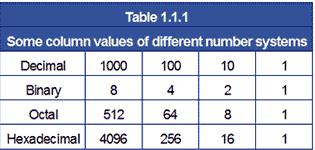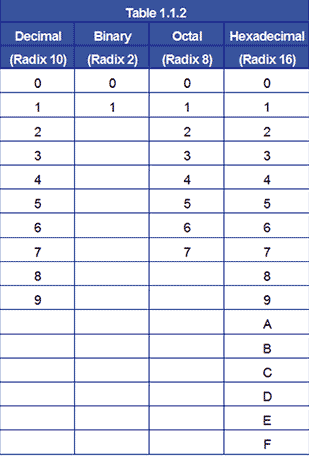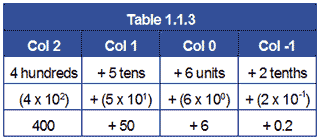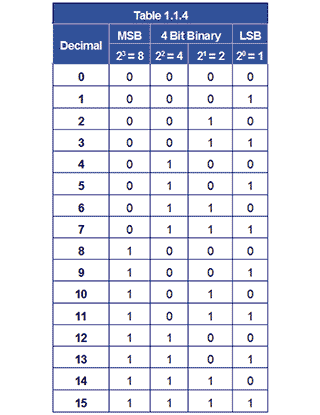Courses

# Number Systems Electrical Engineering (EE) Notes | EduRev

## Electrical Engineering (EE) : Number Systems Electrical Engineering (EE) Notes | EduRev

The document Number Systems Electrical Engineering (EE) Notes | EduRev is a part of the Electrical Engineering (EE) Course Digital Electronics.
All you need of Electrical Engineering (EE) at this link: Electrical Engineering (EE)

## Number Systems

Most number systems follow a common pattern for writing down the value of a number:

A fixed number of values can be written with a single numerical character, then a new column is used to count how many times the highest value in the counting system has been reached. The number of numerical values the system uses is called the base of the system. For example, the decimal system has 10 numerical characters and so has a base of 10:

0 1 2 3 4 5 6 7 8 9

For writing numbers greater than 9 a second column is added to the left, and this column has 10 times the value of the column immediately to its right.

Because number systems commonly used in digital electronics have different base values to the decimal system, they look less familiar, but work in essentially the same way.

### Decimal, (base 10)

Decimal has ten values 0 to 9. If larger values than 9 are needed, extra columns are added to the left. Each column value is ten times the value of the column to its right. For example the decimal value twenty-two is written 22 (2 tens + 2 ones).

### Binary, (base 2)

Binary has only two values 0 and 1. If larger values than 1 are needed, extra columns are added to the left. Each column value is now twice the value of the column to its right. For example the decimal value three is written 11 in binary (1 two + 1 one).

### Octal, (base 8)

Octal has eight values 0 to 7. If larger values than 7 are needed, extra columns are added to the left. Each column value is now 8 times the value of the column to its right. For example the decimal value twenty-seven is written 33 in octal (3 eights + 3 ones).

Hexadecimal has sixteen values 0 to 15, but to keep all these values in a single column, the 16 values (0 to 15) are written as 0 to F, using the letters A to F to represent numbers 10 to 15, so avoiding the use of a second column. Again, if higher values than 15 (F in hexadecimal) are needed, extra columns to the left are used. Each column value is sixteen times that of the column to its right. For example the decimal value sixty-eight is written as 44 in hexadecimal (4 sixteens + 4 ones).

Each of these different number systems works in the same way, it is just that each system has a different base, and the column values in each system increase by multiples of the base number as columns are added to the left.Because this module describes several different number systems, it is important to know which system is being described. Therefore if there is some doubt which system a number is in, the base of the system, written as a subscript immediately after the value, is used to identify the number system.

### For example:1010 represents the decimal value ten. (1 ten + 0 units)

102 represents the binary value two. (1 two + 0 units)

108 represents the octal value eight. (1 eight + 0 units)

1016 represents the hexadecimal value sixteen. (1 sixteen + 0 units)

The base of a system, more properly called the RADIX, is the number of different values that can be expressed using a single digit. Therefore the decimal system has a radix of 10, the octal system has a radix of 8, hexadecimal is radix 16, and binary radix 2                         .The range of number values in different number systems is shown in Table 1.1.2, Notice that because the hexadecimal system must express 16 values using only one column, it uses the letters A B C D E & F to represent the numbers 10 to 15.

When writing a number, the digits used give its value, but the number is ‘scaled’ by its RADIX POINT.

For example, 456.210 is ten times bigger than 45.6210 although the digits are the same.

Notice also that when using multiple number systems, the term ‘RADIX point’ instead of ‘DECIMAL point’ is used. When using decimal numbers, a decimal point is used, but if a different system is used, it would be wrong to call the point a decimal point, it would need to be called "Binary point" or "Octal point" etc. The simplest way around this is to refer to the point in any system (which will of course have its value labelled with its radix) as the RADIX POINT.

## Exponents

A decimal number such as 456.210 can be considered as the sum of the values of its individual digits, where each digit has a value dependent on its position within the number (the value of the column):

= 456.210Each digit in the number is multiplied by the system radix raised to a power depending on its position relative to the radix point. This is called the EXPONENT. The digit immediately to the left of the radix point has the exponent 0 applied to its radix, and for each place to the left, the exponent increases by one. The first place to the right of the radix point has the exponent -1 and so on, positive exponents to the left of the radix point and negative exponents to the right.

This method of writing numbers is widely used in electronics with decimal numbers, but can be used with any number system. Only the radix is different.

Hexadecimal exponents 98.216 = (9 x 161) + (8 x 160) + (2 x 16-1)

Octal exponents 56.28 = (5 x 81) + (6 x 80) + (2 x 8-1)

Binary Exponents 10.12 = (1 x 21) + (0 x 20) + (1 x 2-1)

When using your calculator for the above examples you may find that it does not like radix points in anything other than decimal mode. This is common with many electronic calculators.

## Floating Point Notation

If electronic calculators cannot use radix points other than in decimal, this could be a problem. Fortunately for every problem there is a solution. The radix exponent can also be used to eliminate the radix point, without altering the value of the number. In the example below, see how the value remains the same while the radix point moves. It is all done by changing the radix exponent.

102.610 = 102.6 x 100 = 10.26 x 101 = 1.026 x 102 = .1026 x 103

The radix point is moved one place to the left by increasing the exponent by one.

It is also possible to move the radix point to the right by decreasing the exponent. In this way the radix point can be positioned wherever it is required, in any number system, simply by changing the exponent. This is called FLOATING POINT NOTATION and it is how calculators handle decimal points in calculations.

## Normalised Form

By putting the radix point at the front of the number, and keeping it there by changing the exponent, calculations become easier to do electronically, in any radix.

## Electronic storage of numbers.

A number written (or stored) in this way, with the radix point at the left of the most significant digit is said to be in NORMALISED FORM. For example .110112 x 23 is the normalised form of the binary number 110.112. Because numbers in electronic systems are stored as binary digits, and a binary digit can only be 1 or 0, it is not possible to store the radix point within the number. Therefore the number is stored in its normalised form and the exponent is stored separately. The exponent is then reused to restore the radix point to its correct position when the number is displayed.

In electronics systems a single binary digit is called a bit (short for Binary DigIT), but as using a single digit would seriously limit the maths that could be performed, binary bits are normally used in groups.

4 bits = 1 nibble

8 bits = 1 byte

Multiple bytes, such as 16 bits, 32 bits, 64 bits are usually called ‘words’, e.g. a 32 bit word. The length of the word depends on how many bits can be physically handled or stored by the system at one time.

### 4 Bit Binary RepresentationWhen a number is stored in an electronic system, it is stored in a memory location having a fixed number of binary bits. Some of these memory locations are used for general storage whilst others, having some special function, are called registers. Wherever a number is stored, it will be held in some form of binary, and must always have a set number of bits. Therefore a decimal number such as 13, which can be expressed in four binary bits as 11012 becomes 000011012 when stored in an eight-bit register. This is achieved by adding four NON SIGNIFICANT ZEROS to the left of the most significant ‘1’ digit.

Using this system, a binary register that is n bits wide can hold 2n values.

Therefore an 8 bit register can hold 28 values = 256 values (0 to 255)

A 4 bit register can hold 24 values = 16 values (0 to 15)

Offer running on EduRev: Apply code STAYHOME200 to get INR 200 off on our premium plan EduRev Infinity!

## Digital Electronics

92 videos|91 docs|12 tests

,

,

,

,

,

,

,

,

,

,

,

,

,

,

,

,

,

,

,

,

,

;